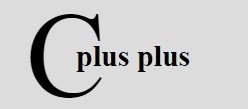# C++ Basic Programs

Here are some basic C++ programs listed to understand the basic concept of C++ programming. In last, some intermediate level C++ programs also listed. You can call those programs as basic C++ program of intermediate level. Let's start with basic C++ programs.

## C++ Basic Program No. 1

```/* C++ Basic Programs */

#include<iostream.h>
#include<conio.h>
void main()
{
clrscr();
cout<<"This is basic C++ program.";
getch();
}```

Here is the sample output of the above C++ program: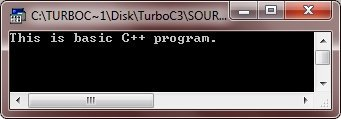## C++ Basic Program No. 2

Here is another type of basic program in C++

```/* C++ Basic Programs */

#include<iostream.h>
#include<conio.h>
void main()
{
clrscr();
char ch;
cout<<"Want to learn C++ ? (y/n).. ";
cin>>ch;
if(ch=='y' || ch=='Y')
{
cout<<"\nWelcome to codescracker.com";
cout<<"\nYou can learn all about C++ here\n";
cout<<"From very basic to an advance level\n";
}

getch();
}```

Here is the sample run of the above C++ program: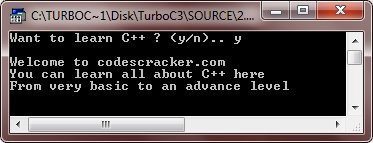## C++ Basic Program No. 3

Let's take another type of basic program in C++

```/* C++ Basic Programs */

#include<iostream.h>
#include<conio.h>
void main()
{
clrscr();
int counter, i;
int num;
cout<<"Initializing counter...\n";
cout<<"Enter a number: ";
cin>>num;
cout<<"\n";
for(i=num; i>=0; i--)
{
cout<<i<<"  ";
}
cout<<"Fire...!!";

getch();
}```

Below is the sample run of the above C++ program: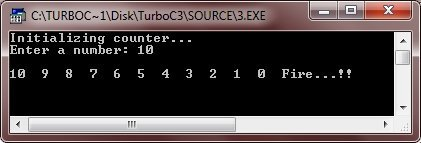## C++ Basic Program No. 4

Below is another C++ basic program of other type. This program is the imporoved version of the above C++ program.

```/* C++ Basic Programs */

#include<iostream.h>
#include<conio.h>
#include<dos.h>
void main()
{
clrscr();
int counter, i;
int num;
cout<<"Initializing counter...\n";
cout<<"Enter a number: ";
cin>>num;
cout<<"\n";
for(i=num; i>=0; i--)
{
cout<<i<<"  ";
delay(500);
}
cout<<"Fire...!!";

getch();
}```

Here is the sample run of the above C++ program.: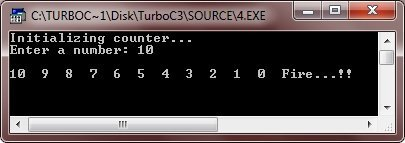## C++ Basic Program No. 5

Here is another type of basic program in C++.

```/* C++ Basic Programs */

#include<iostream.h>
#include<conio.h>
void main()
{
clrscr();
int check;
cout<<"Enter 5: ";
cin>>check;
if(check==5)
{
cout<<"What an honest programmer, you are..!!";
}
else
{
cout<<"Sorry..!!..You entered wrong..!!";
}
getch();
}```

Here are the two sample runs of the above C++ program: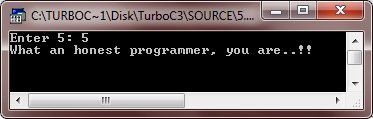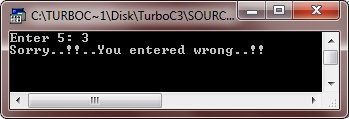## C++ Basic Program No. 6

Let's take another basic program in C++

```/* C++ Basic Programs */

#include<iostream.h>
#include<conio.h>
#include<stdlib.h>
void main()
{
clrscr();
char inf;
char ans, ch;
do
{
cout<<"Enter anything... ";
cin.get(inf, 20);
cin.get(ch);
cout<<"\nYou entered "<<inf<<endl;
cout<<"\nWant to enter more ? (y/n).. ";
cin>>ans;
cin.get(ch);
cout<<"\n";
}while(ans=='y' || ans=='Y');
getch();
}```

Below is the sample run of this C++ program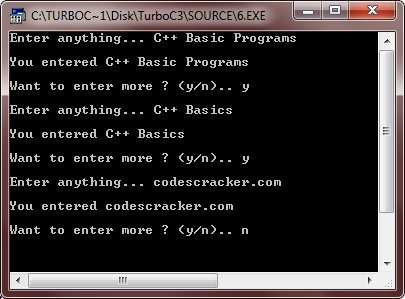## C++ Basic Program No. 7

Let's take another type of C++ basic program.

```/* C++ Basic Programs */

#include<iostream.h>
#include<conio.h>
#include<stdlib.h>
void main()
{
clrscr();
int star, counter=0;
int i, j;
char check;
cout<<"How many start you want to print ? ";
cin>>star;
counter=star;
cout<<"\nWant to print in diagonal form ? (y/n).. ";
cin>>check;
if(check=='y' || check=='Y')
{
for(i=0; i<star; i++)
{
for(j=0; j<i; j++)
{
counter--;
if(counter<0)
{
getch();
exit(1);
}
else
{
cout<<"* ";
}
}
cout<<"\n";
}
}
else
{
cout<<"\n";
for(i=0; i<star; i++)
{
cout<<"* ";
}
}
getch();
}```

Below are the two sample runs of the above C++ program: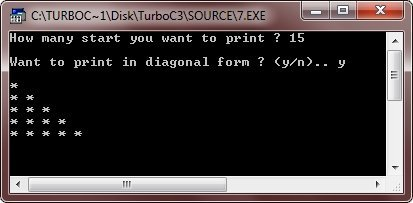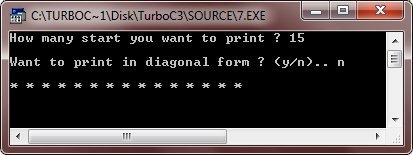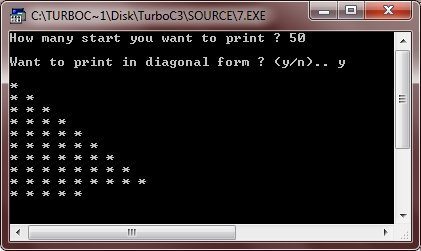## C++ Basic Program No. 8

Let's take one more type of C++ basic program.

```/* C++ Basic Programs */

#include<iostream.h>
#include<stdlib.h>
#include<conio.h>
void startup(void);
void subtract(int, int);
void multiply(int, int);
void divide(int, int);
void main()
{
clrscr();
startup();
int num1, num2;
char ch;
do
{
cout<<"\n2. Subtract";
cout<<"\n3. Multiply";
cout<<"\n4. Divide";
cout<<"\n5. Exit\n";
cout<<"\nWhat do you want ? ";
cin>>ch;
if(ch>='1' && ch<='4')
{
cout<<"\nEnter any two number: ";
cin>>num1>>num2;
}
switch(ch)
{
case '1':
break;
case '2':
subtract(num1, num2);
break;
case '3':
multiply(num1, num2);
break;
case '4':
divide(num1, num2);
break;
case '5':
exit(1);
default:
cout<<"Wrong choice..!!..Press a key to exit..";
getch();
exit(2);
}
cout<<"\n";
} while(ch>='1' && ch<='5');

getch();
}

void startup(void)
{
cout<<"Welcome to codescracker.com\n";
cout<<"You are at C++ Basic Programs\n";
cout<<"Press any key to start:\n";
getch();
}

{
cout<<"Result = "<<a+b;
}

void subtract(int a, int b)
{
cout<<"Result = "<<a-b;
}

void multiply(int a, int b)
{
cout<<"Result = "<<a*b;
}

void divide(int a, int b)
{
if(b==0)
{
cout<<"Divide by zero error..!!";
cout<<"\nPress any key to exti..\n";
getch();
exit(3);
}
cout<<"Result = "<<a/b;
}```

Here are some sample runs of the above C++ program: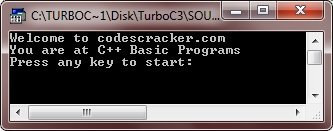Now press any key to start working. After pressing any key, here are some sample runs.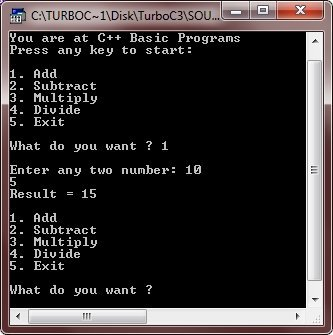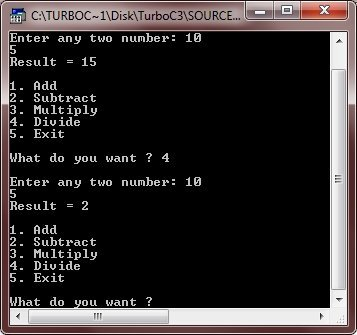### More C++ Basic Programs

Here are some more C++ basic programs listed, that you can go for:

Tools
Calculator